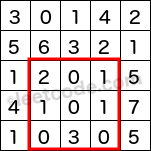## Range Sum Query 2D - Immutable

07/17/2016 Dynamic Programming

## Question

Given a 2D matrix matrix, find the sum of the elements inside the rectangle defined by its upper left corner (row1, col1) and lower right corner (row2, col2).The above rectangle (with the red border) is defined by (row1, col1) = (2, 1) and (row2, col2) = (4, 3), which contains sum = 8.

Example:

Given matrix = [
[3, 0, 1, 4, 2],
[5, 6, 3, 2, 1],
[1, 2, 0, 1, 5],
[4, 1, 0, 1, 7],
[1, 0, 3, 0, 5]
]

sumRegion(2, 1, 4, 3) -> 8
sumRegion(1, 1, 2, 2) -> 11
sumRegion(1, 2, 2, 4) -> 12


Note:

1. You may assume that the matrix does not change.
2. There are many calls to sumRegion function.
3. You may assume that row1 ≤ row2 and col1 ≤ col2.

## Solution

Result: Accepted Time: ms

Here should be some explanations.

class NumMatrix {
public:
vector<vector<int>> vect;
NumMatrix(vector<vector<int>> &mat) {
if(mat.size() == 0) return ;
vect = vector<vector<int>>(mat.size()+1,vector<int>(mat.size()+1,0));
for(int i = 0; i < mat.size(); i++)
for(int j = 0; j < mat.size(); j++)
vect[i+1][j+1] = vect[i+1][j] + mat[i][j];
for(int i = 0; i < mat.size(); i++)
for(int j = 0; j < mat.size(); j++)
vect[i+1][j+1] += vect[i][j+1];

}

int sumRegion(int row1, int col1, int row2, int col2) {
return vect[row2+1][col2+1] + vect[row1][col1] - vect[row2+1][col1] - vect[row1][col2+1];

}
};

// Your NumMatrix object will be instantiated and called as such:
// NumMatrix numMatrix(matrix);
// numMatrix.sumRegion(0, 1, 2, 3);
// numMatrix.sumRegion(1, 2, 3, 4);


Complexity Analytics

• Time Complexity: $O(n^2)$
• Space Complexity: $O(n^2)$